# Part 1: Introduction to Vectors | Beginner’s Guide to Year 12 Ext 1 Maths

Struggling to get momentum with Ext 1 maths? In this article, we give you an introduction to Vectors and their representation so you can get going in the right direction!Feeling a bit directionless when studying vectors? In this article, we give you a comprehensive introduction to vectors for Year 12 Maths Ext 1 so you can find your way to a Band 6.

## Introduction to Vectors

• The NESA syllabus outcomes
• What a Vector Is
• How Vectors are Represented.
• Different types of vectors, including unit, position and displacement vectors
• Addition, subtraction and scalar multiplication of vectors, algebraically and geometrically

## Year 12 Mathematics Extension 1: Introduction to Vectors and their representation

Understanding how vectors operate plays a crucial role in visualising basic operations such as addition, subtraction and multiplication on a two-dimensional plane, which will then enable students to solve and prove harder geometric properties and applications.

## NESA Syllabus Outcomes

The NESA syllabus outcomes for Year 12 Maths Extension 1 students are:

• Define a vector quantity as a quantity having both magnitude and direction, and examine examples of vectors, including displacement and velocity:
• Explain the distinction between a position vector and a displacement (relative vector)
• Define and use a variety of notations and representations for vectors in two dimensions:
• Use standard notations for vectors, for example: $$\stackrel{\rm a}{\rm \text{~}}, \vec{(AB)}, \boldsymbol{a}$$
• Represent vectors graphically in two dimensions as directed line segments
• Define unit vectors as vectors of magnitude 1, and the standard two-dimensional perpendicular unit vectors $$i$$ and $$j$$
• Express and use vectors in two dimensions in a variety of forms, including component form, ordered pairs and column vector notation.
• Perform addition and subtraction of vectors and multiplication of a vector and a scalar algebraically and geometrically, and interpret these operations in geometric terms:
• Graphically represent a scalar multiple of a vector
• Use the triangle law and the parallelogram law to find the sum and difference of two vectors
• Define and use addition and subtraction of vectors in component form.
• Define and use multiplication by a scalar of a vector in component form.

## Assumed Knowledge

Students should already be familiar with basic algebraic operations, geometry, trigonometry and coordinate geometry.

## What is a Vector?

A vector is a quantity which has both magnitude and direction. For example, a force of 60 Newtons is applied to the ground. This means that the force’s magnitude is 60 and its direction is downwards, towards the ground.

A vector can be denoted using boldface, $$\bf{u}$$, an arrow above the variable, $$\vec{u}$$, or a tilde under the variable, $$\stackrel{\rm u}{\rm \text{~}}$$. A vector running from point $$A$$ to point $$B$$ is denoted by $$\vec{(AB)}$$.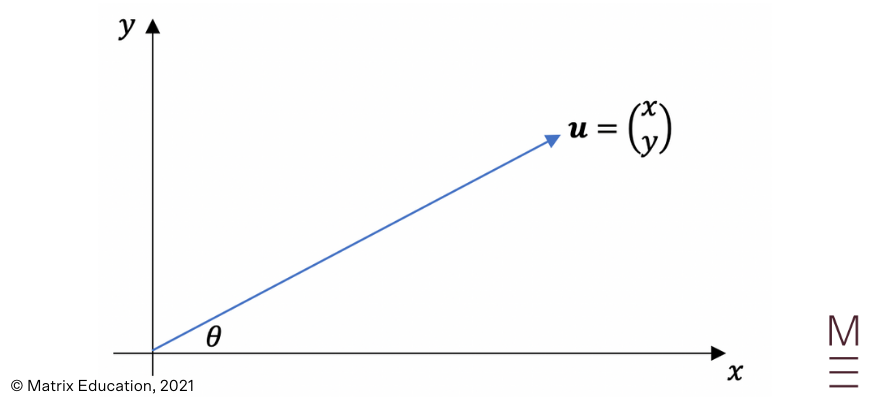The diagram above represents vector u, which is simply an arrow: The diagram above represents vector $$\bf{u}$$, which is simply an arrow:  The magnitude of $$\bf{u}$$, denoted $$|\bf{u}|$$, is the length of the arrow.  The direction of $${\bf u}$$, denoted $$θ$$, is the direction that the arrow is pointing towards.  The pointy end of the arrow is called the head, and the other end is called the tail.

Vectors can also be represented algebraically by placing them in the ($$x,y$$) plane:

$${\bf u} \ = \left(\ _y^x \right) \ = (x,y)$$
• The $$x$$ value is called the x-component, and the $$y$$ value is called the y-component.
• $${\bf u}=\left(_y^x\right)$$ is called the column vector form.
• $${\bf u}=(x,y)$$ is called the Cartesian form.

Example 1:

Which of the following is the correct notation of a vector?

$$\vec{(AB)}, \ {\bf u}, \ V_4, \ OP, \ \stackrel{\rm m}{\rm \text{~}}$$

Solution 1:

$$\vec{(AB)}, \ , {\bf u}, \ \stackrel{\rm m}{\rm \text{~}}$$

Example 2:

Express each vector in the diagram below in column vector and Cartesian forms: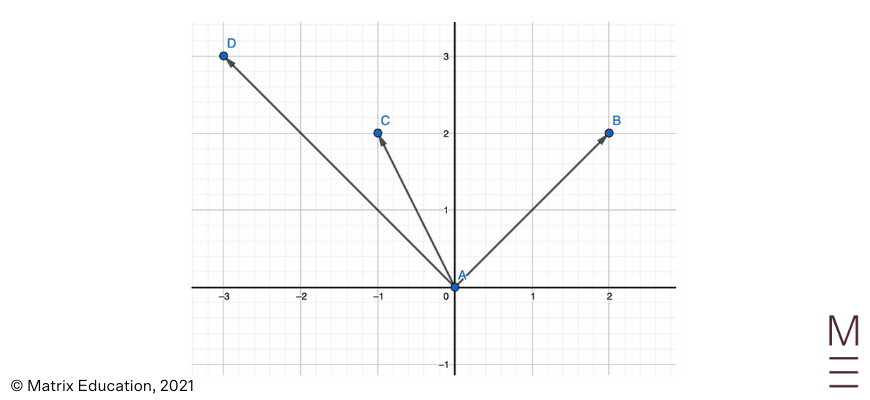Vector Column vector form Cartesian form $$\vec{AB}$$ $$\left({2}\atop{2}\right)$$ $$(2,2)$$ $$\vec{AC}$$ $$\left( {-1} \atop {2} \right)$$ $$(-1,2)$$ $$\vec{AD}$$ $$\left( {-3} \atop {2} \right)$$ $$(-3,3)$$

## Put your knowledge of vectors to the test!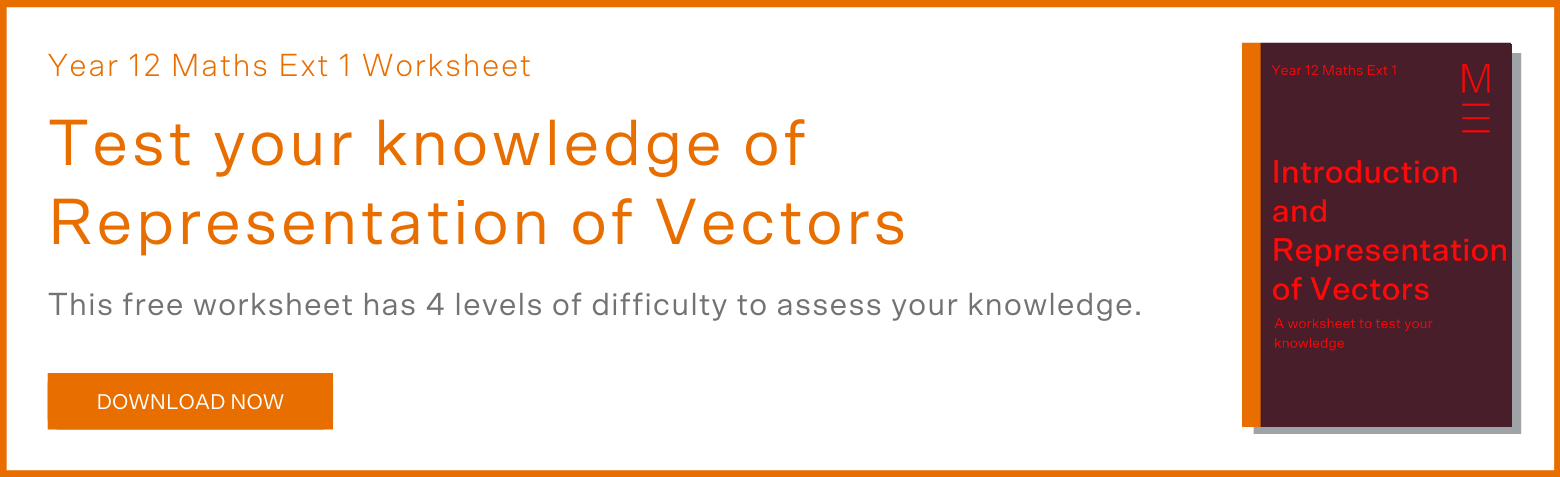## Unit Vectors, Position Vectors and Displacement Vectors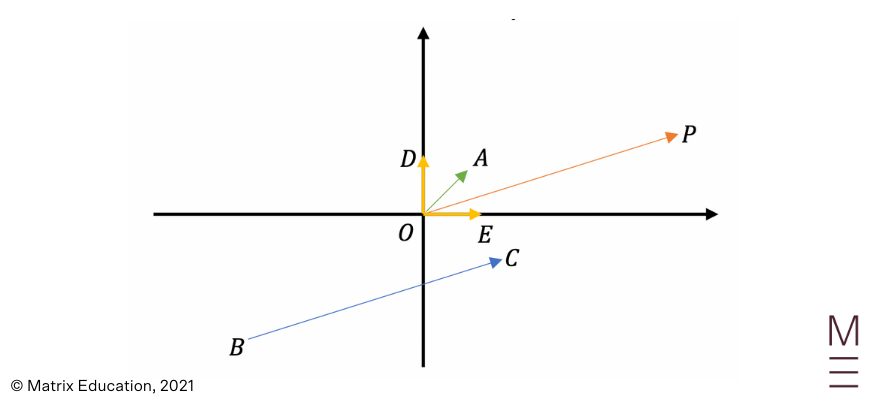The diagram above contains unit vectors, a position vector, a displacement vector, as well as the standard two-dimensional perpendicular unit vectors:

• A unit vector is a vector of magnitude one. The unit vector in the diagram above is $$\vec{OA}$$ (green).
• A position vector is a vector whose initial point is bounded by the origin, $$O$$. The position vectors in the diagram above are $$\vec{OA}, \vec{OP}, \vec{OD}$$ and $$\vec{OE}$$.
• A displacement vector is a vector which can move freely around the two-dimensional plane. Its point of origin is unimportant, as we are only interested in its magnitude and direction. The displacement vector in the diagram above is $$\vec{BC}$$.
• The vectors $$\vec{OP}$$ and $$\vec{BC}$$ are equal because they have the same magnitude (length) and pointing in the same direction.
• The standard two-dimensional perpendicular unit vectors denoted $$\stackrel{\rm i}{\rm \text{~}}$$ and $$\stackrel{\rm j}{\rm \text{~}}$$, have magnitude one. $$\stackrel{\rm i}{\rm \text{~}}$$ is parallel to the x-axis and $$\stackrel{\rm m}{\rm \text{~}}$$ is parallel to the y-axis. In the diagram above, $$\stackrel{\rm i}{\rm \text{~}}$$ is $$\vec{OE}$$ and $$\stackrel{\rm j}{\rm \text{~}}$$ is $$\vec{OD}$$.

Using the standard two-dimensional perpendicular unit vectors, we can represent vectors in a third way, the component form. Knowing that $${\bf i} = \left( {1}\atop{0}\right)$$ and $${\bf j} = \left( {1}\atop{0}\right)$$, the vector $${\bf u}$$ can be expressed as:

$${\bf u} = \left( {x}\atop{y}\right)=(x,y)=x{\bf i}+y{\bf j}$$

The coefficient of $${\bf i}$$ is called the x-component, and the coefficient of $${\bf j}$$ is called the y-component.

Example 1: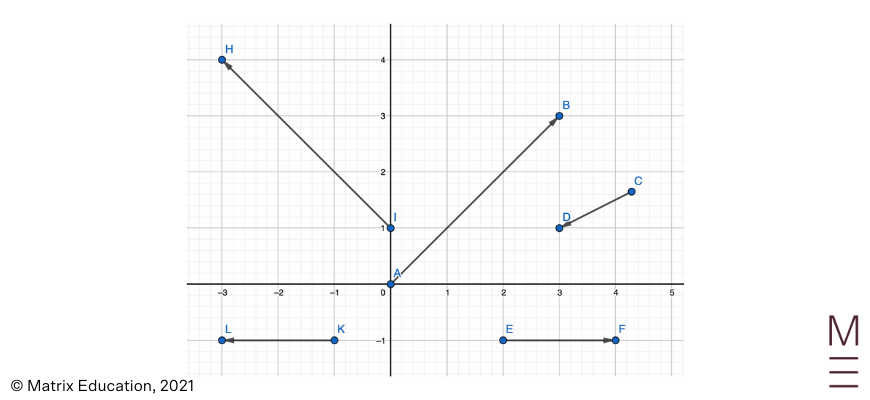1. List the position vectors from the diagram.
2. List the position vectors from the diagram.
3. List the displacement vectors from the diagram.
4. Which two pairs of vectors have the same magnitude?
5. Are any vectors equal to each other? (Remember that vectors are equal if they have the same magnitude and direction)
6. Express $$\vec{AB}$$ in component form.

Solution 1:

1. $$\vec{AB}$$
2. $$\vec{CD}, \vec{EF}, \vec{KL}, \vec{IH}$$
3. $$\vec{AB}$$ and $$\vec{IH}, \vec{EF}$$ and $$\vec{KL}$$
4. No. Even though $$\vec{AB}$$ and $$\vec{IH}, \vec{EF}$$ and $$\vec{KL}$$ have the same magnitude as each other, their directions are different, therefore there are no vectors that are equal to each other.
5. $$\vec{AB} = 3{\bf i} + 3{\bf j}$$

## Addition and Subtraction of vectors

When adding vectors together, we must consider both their magnitude and direction. Vectors are added using the head to tail method:

• Given two vectors, $${\bf u}$$ and $${\bf v}$$ as per below: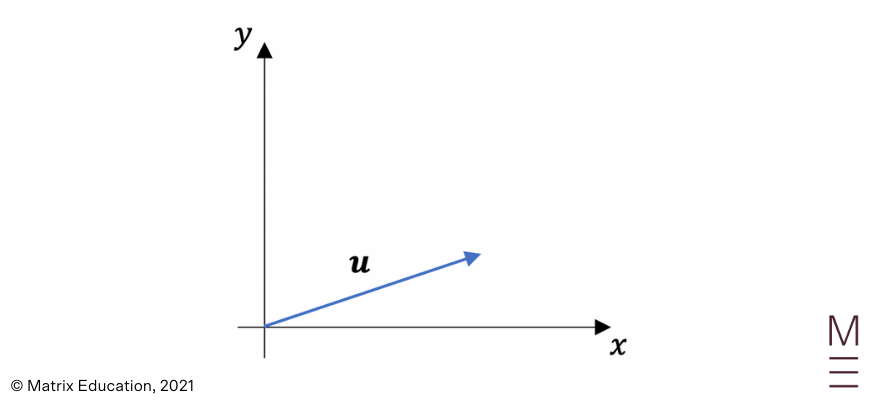and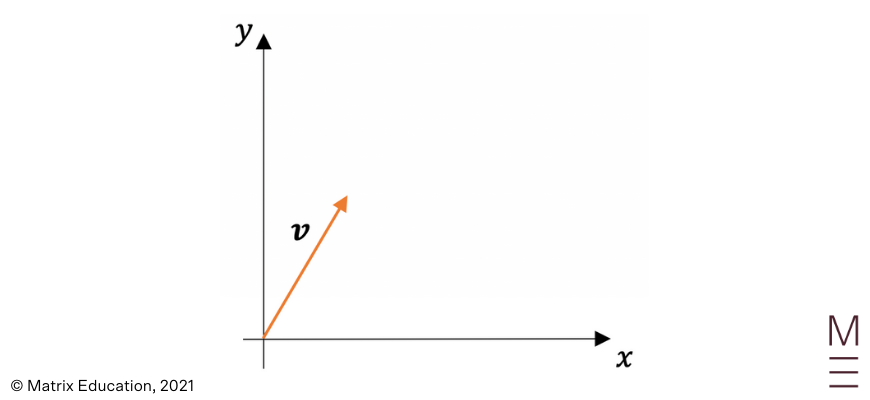• On a new diagram, sketch vector $${\bf u}$$:• Now, lift vector $${\bf v}$$ form the original diagram, positioning its tail at the head of $${\bf u}$$: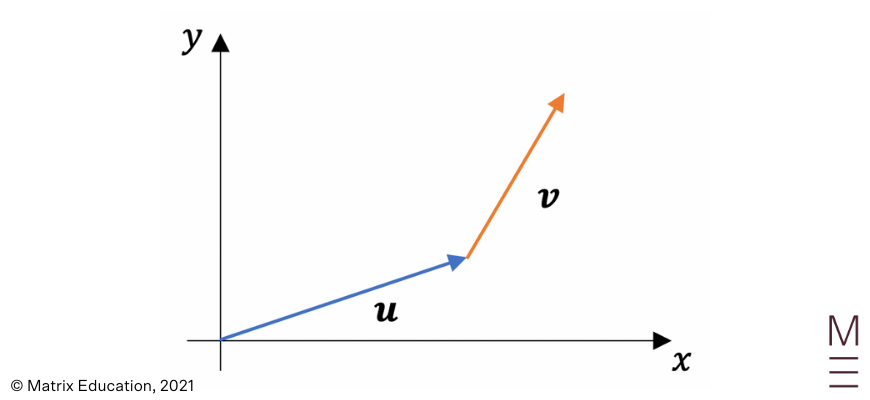• Connect the tail of vector $${\bf u}$$ to the head of vector $${\bf v}$$. This represents the sum of $${\bf u} + {\bf v}$$, which is the resultant vector.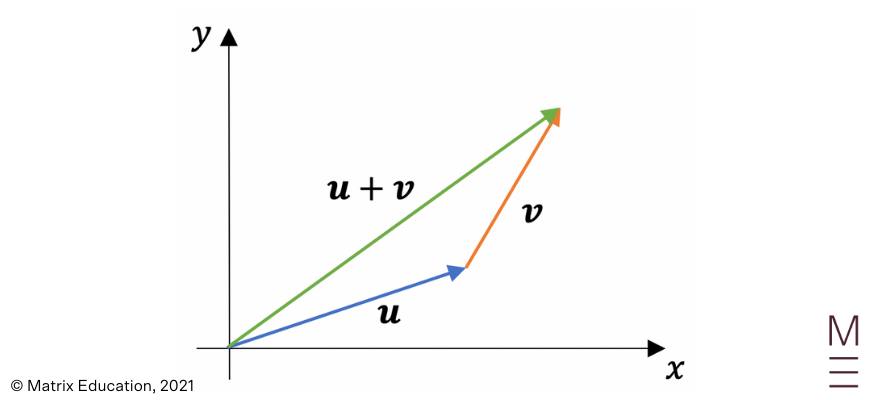Subtracting vectors is the equivalent of adding the negative vector. The negative vector is a vector which has the same magnitude but opposite direction. We can once again use the head to tail method for the subtraction of vectors:

• Given two vectors, $${\bf u}$$ and $${\bf v}$$ as per below:and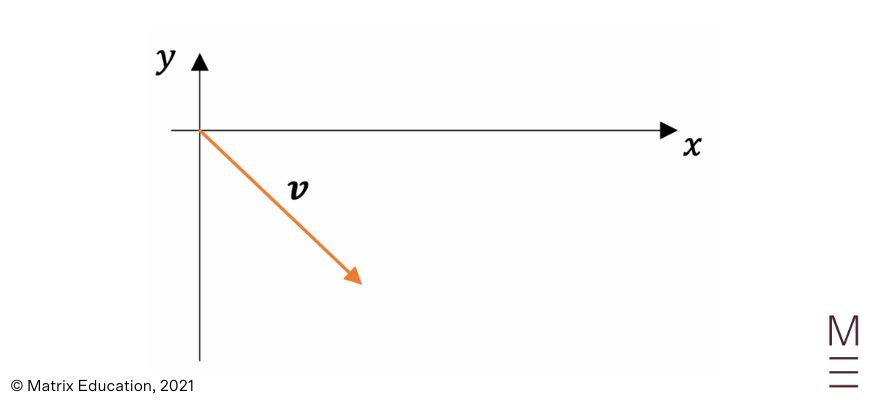• We can obtain the negative of vector $${\bf v}$$ by flipping the direction of the vector: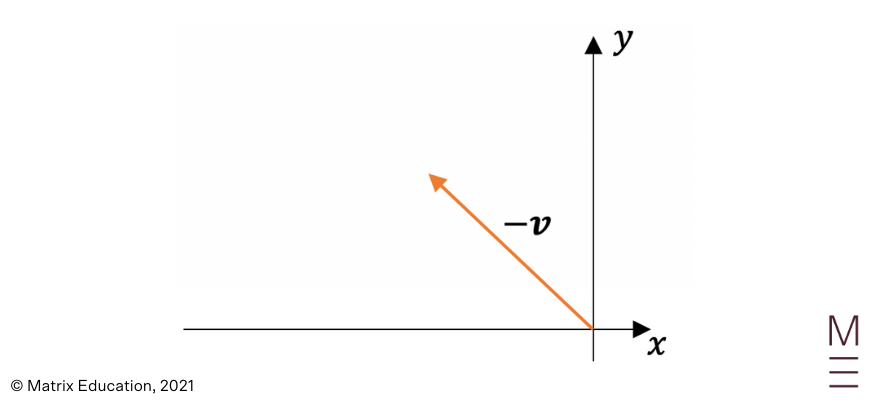• Repeat the same process as for addition, however, we’re adding $${\bf u}$$ and $${\bf -v}$$: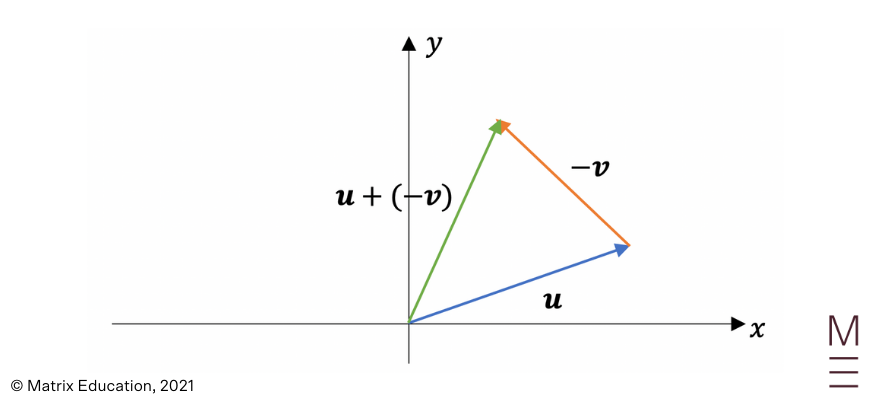• This leads to the triangle law of addition and subtraction, as the process of adding and subtracting vectors forms a triangle, as seen in previous diagrams. Another law associated with the process of adding and subtracting vectors is the parallelogram law of addition and subtraction. This can be illustrated below: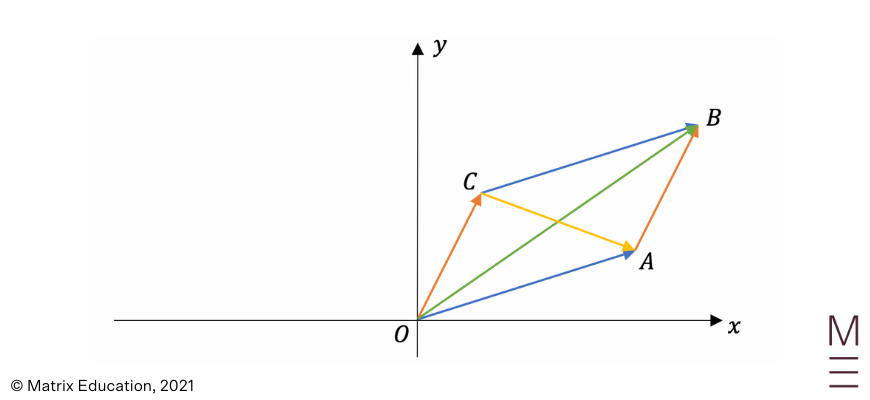From the diagram above, we can see that vectors $$\vec{OA}$$ and $$\vec{CB}$$ are equal to each other, and vectors $$\vec{OC}$$and $$\vec{AB}$$are equal to each other. These four vectors form a parallelogram.
$$\vec{OB}=\vec{OA} + \vec{AB}$$, and $$\vec{CA}=\vec{OA} -\vec{OC}$$, which are the diagonals of the parallelogram.

Example 1:

Given vectors $${\bf a}$$ and $${\bf b}$$ as below: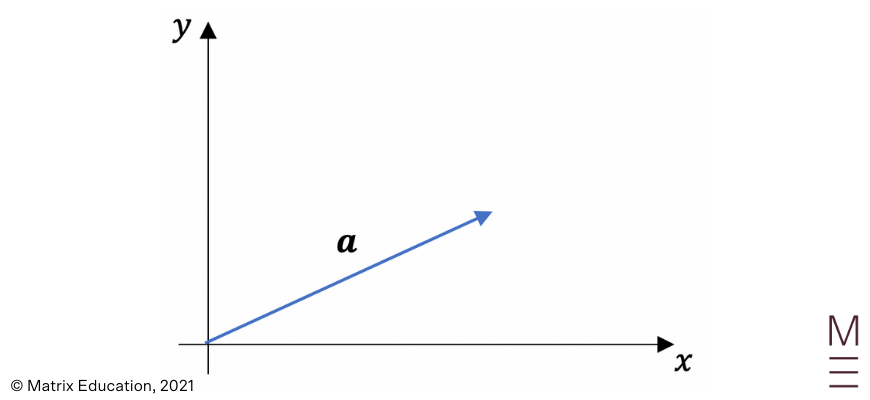and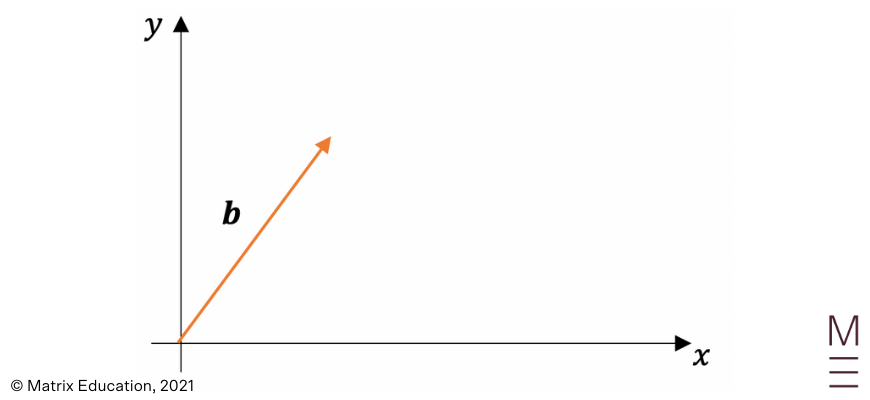Use the given information to draw the following vectors:

a) $${\bf a}+{\bf b}$$
b) $${\bf a}-{\bf b}$$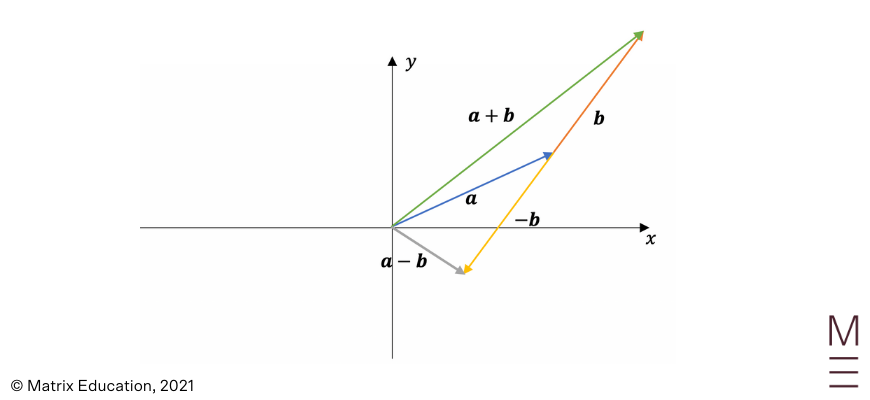## Scalar multiplication of vectors

• A scalar multiple of vector $${\bf v}$$ is any vector $$α{\bf v}$$, where $$α$$ is the scaling factor.
• Taking a scalar multiple of a vector means scaling or shrinking that vector by the scaling factor:
• If the scaling factor, $$α>0$$, then $$α{\bf v}$$ will have the same direction as $${\bf v}$$.
• If the scaling factor, $$α<0$$, then $$α{\bf v}$$ will have the opposite direction to $${\bf v}$$.
• If the scaling factor, $$α>1$$, then $$α{\bf v}$$ will be larger than $${\bf v}$$.
• If the scaling factor, $$0<α<1$$, then $$α{\bf v}$$ will be smaller than $${\bf v}$$.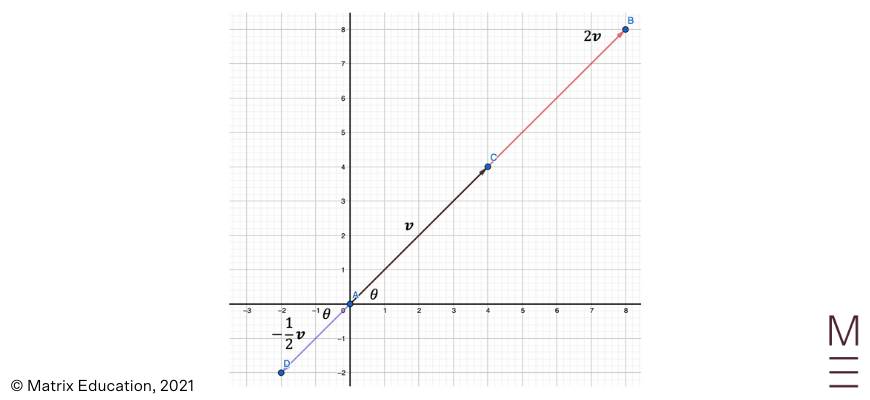Example 1:

Given vectors $${\bf v}$$ and $${\bf v}$$ as per below: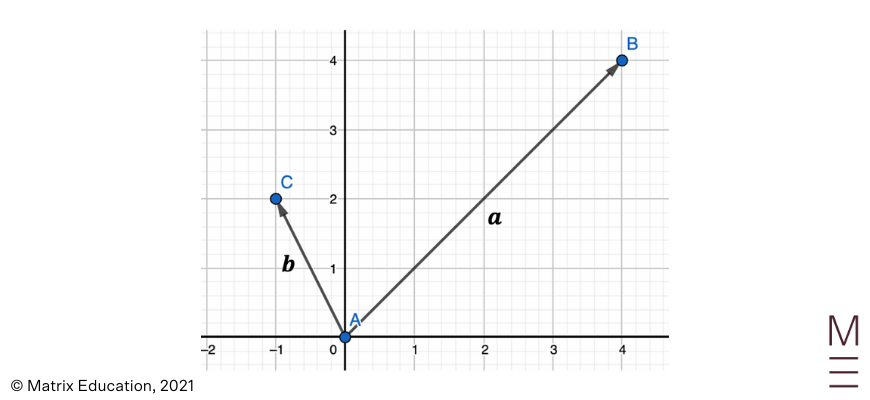Use the given information to draw the following vectors:

a)$$\frac{1}{2} {\bf a}$$
b)$$-3{\bf b}$$
c) $$\frac{1}{2} {\bf a} -3{\bf b}$$

Solution 1: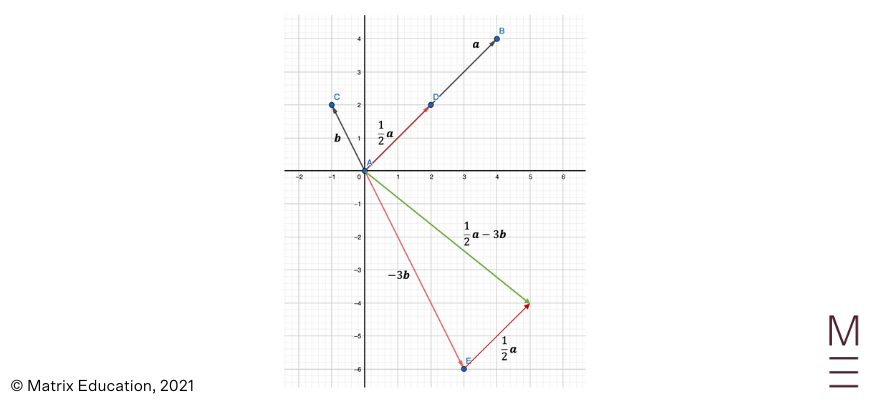## Concept Check Questions

1. Given vectors $${\bf a}$$ and $${\bf b}$$ as per below: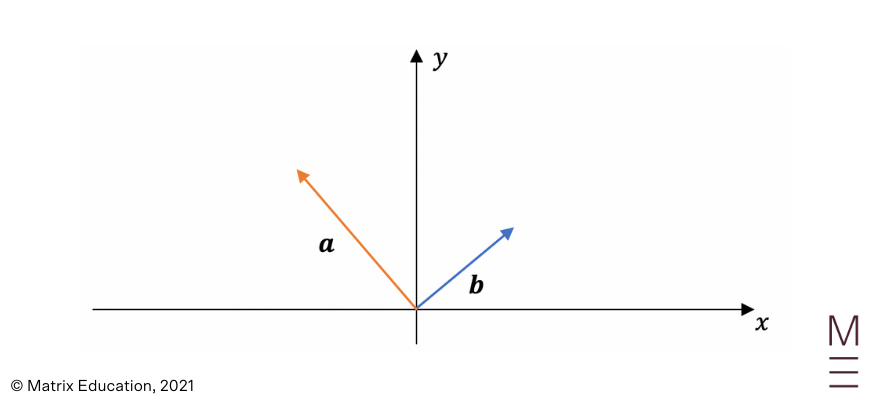Use the given information to draw the following vectors:

1. $${\bf -b}$$
2. $${\bf a} + {\bf b}$$
3. $${\bf a} – {\bf b}$$
4. $${\bf b} – {\bf a}$$

2. Let $$\vec{AB} \ \left( {1}\atop{2}, \right) \vec{AC} = \left( {-2} \atop {3} \right)$$ and $$\vec{AD} \ = \left( {-1} \atop {-4} \right)$$. Draw the following vectors and identify their positions:

1. $$2 \vec{AB}$$
2. $$-3 \vec{AC}$$
3. $$\frac{1}{4} \vec{AD}$$
4. $$2 \vec{AB} + \vec{AC}$$
5. $$\frac{1}{2} \vec{AD} – 2\vec{AC}$$
6. $$\frac{1}{2} \vec{AB} – \frac{1}{3} \vec{AC} + 2 \vec{AD}$$

## Concept Check Solutions

1.

1. $${\bf -b}$$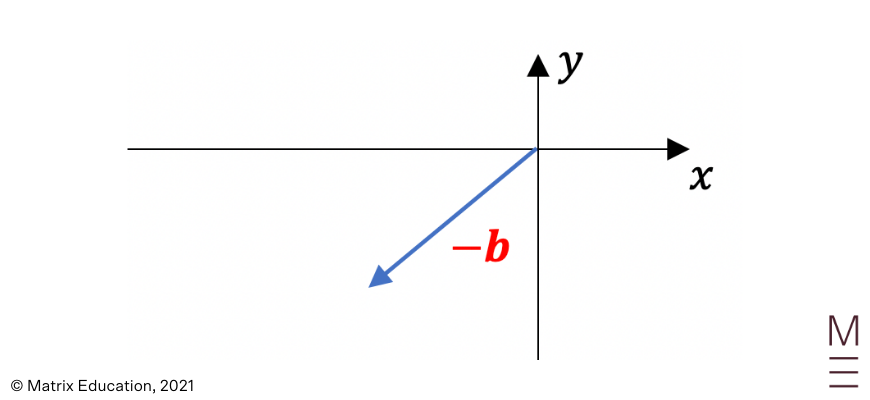2. $${\bf a} + {\bf b}$$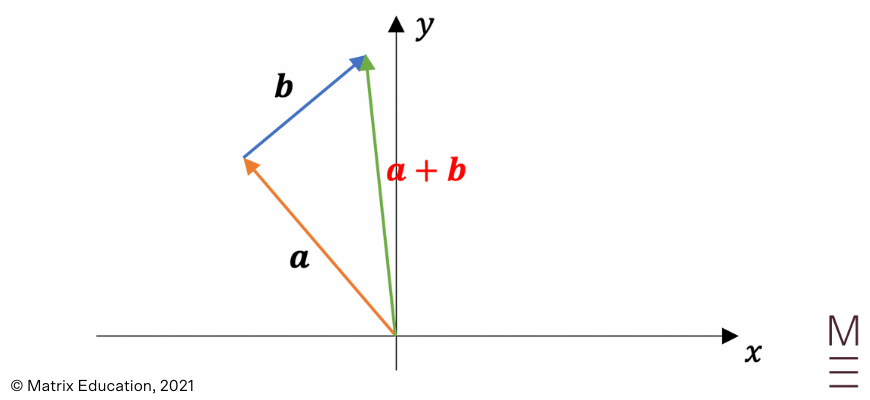3. $${\bf a} – {\bf b}$$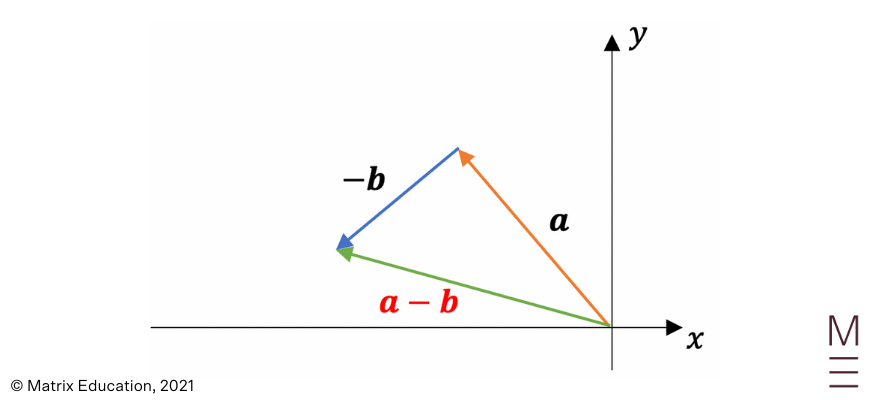4. $${\bf b} – {\bf a}$$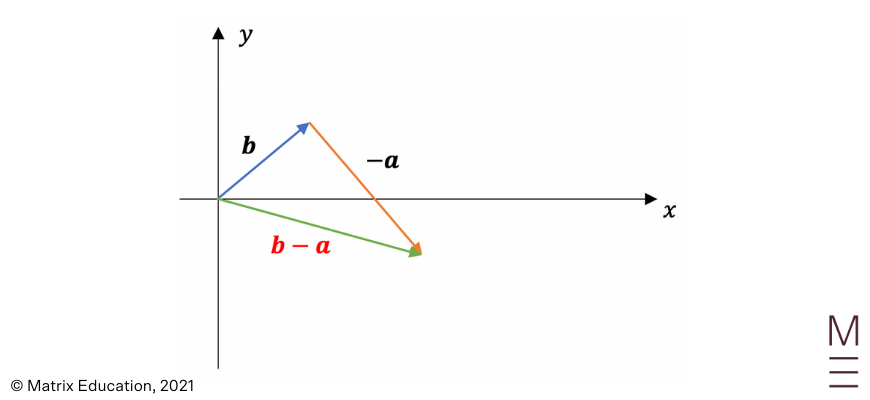2.

In the solutions below, the vectors in red represent the answer

1. $$2 \vec{AB}$$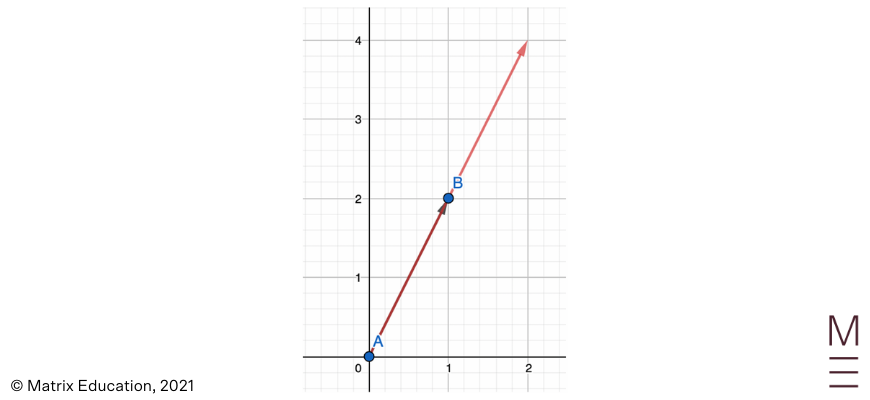2. $$-3 \vec{AC}$$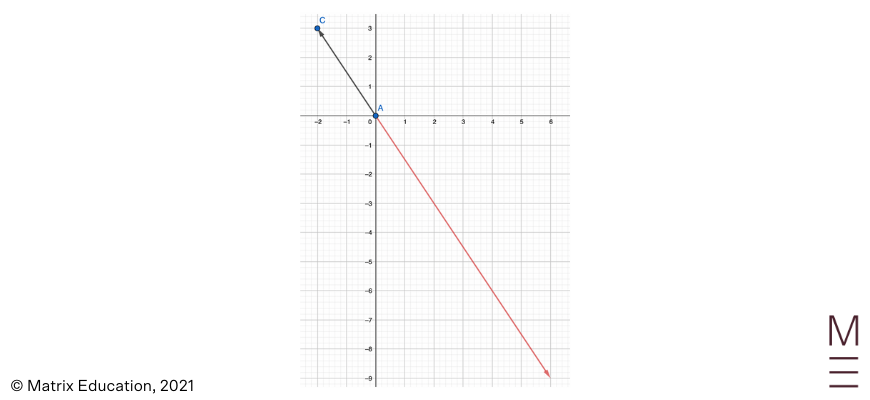3. $$\frac{1}{4} \vec{AD}$$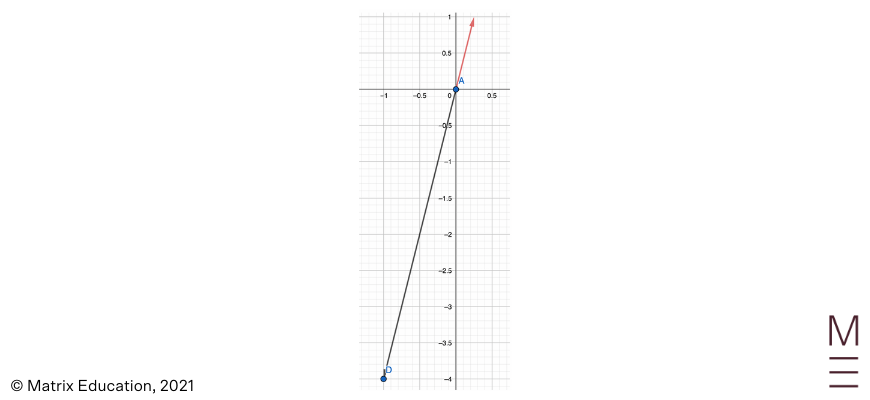4. $$2 \vec{AB} + \vec{AC}$$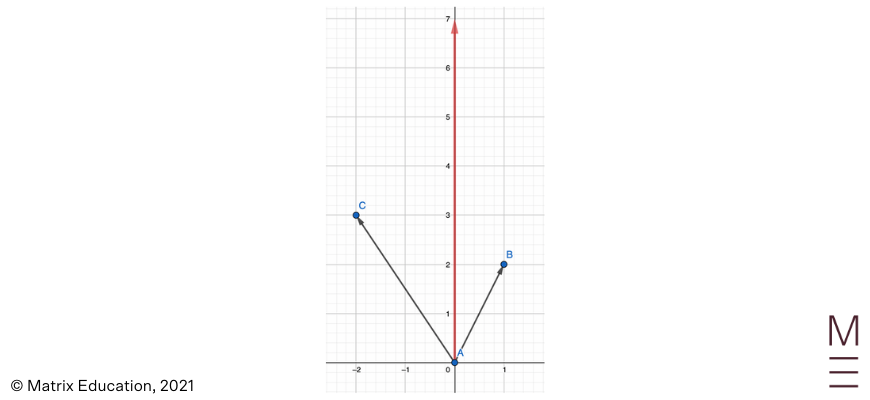5. $$\frac{1}{2} \vec{AD} – 2\vec{AC}$$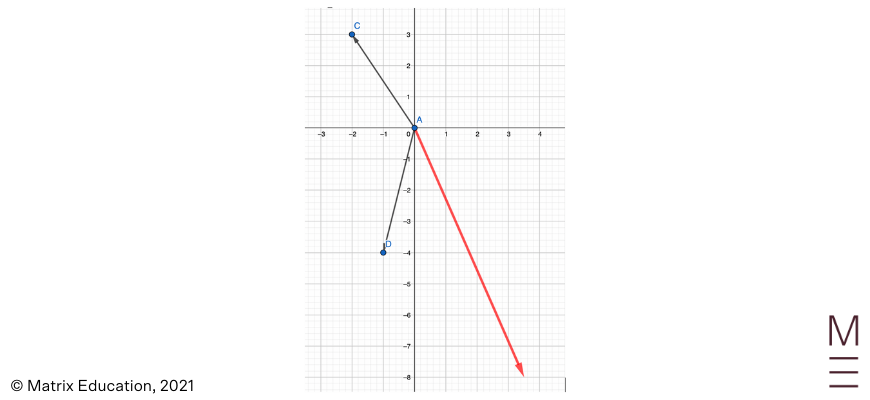6. $$\frac{1}{2} \vec{AB} – \frac{1}{3} \vec{AC} + 2 \vec{AD}$$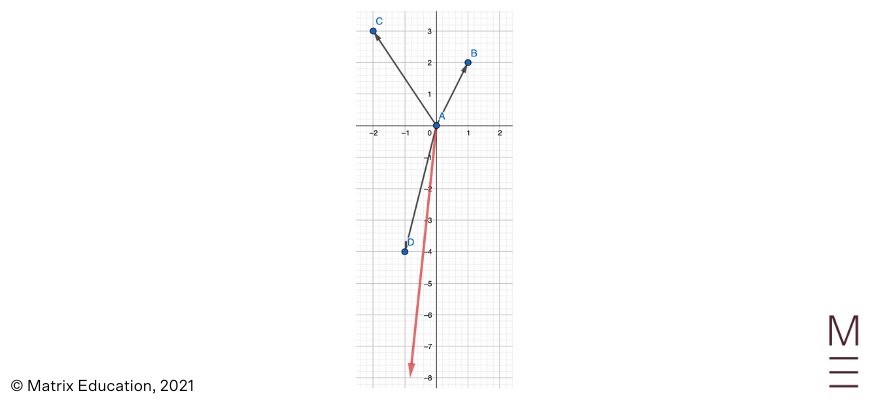## Need to push your marks in the right direction?

The Matrix+ Year 12 Maths Ext 1 online course gives you expert presenters, detailed resources, and Q&A boards wherever you need them. Learn more.© Matrix Education and www.matrix.edu.au, 2021. Unauthorised use and/or duplication of this material without express and written permission from this site’s author and/or owner is strictly prohibited. Excerpts and links may be used, provided that full and clear credit is given to Matrix Education and www.matrix.edu.au with appropriate and specific direction to the original content.